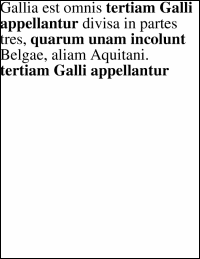AddText FunctionAdds a block of text to the current page.Syntax

 [C#] `int AddText(string text)` [Visual Basic] `Function AddText(text As String) As Integer`Params

 Name Description text The text to be added to the page. return The Object ID of the newly added Text Object.NotesThe following code adds a number of chunks of text to a document. Each chunk is in a different style. This sample makes use of the fact that the Pos is updated to point to the next text insertion point after adding a piece of text. However note that when inserting muti-styled text it is generally more efficient to use the AddTextStyled method. [C#] ```Doc theDoc = new Doc(); theDoc.Page = theDoc.AddPage(); theDoc.FontSize = 48; int theF1 = theDoc.AddFont("Times-Roman"); int theF2 = theDoc.AddFont("Times-Bold"); theDoc.Font = theF1; theDoc.AddText("Gallia est omnis "); theDoc.Font = theF2; theDoc.AddText("tertiam Galli appellantur "); theDoc.Font = theF1; theDoc.AddText("divisa in partes tres, "); theDoc.Font = theF2; theDoc.AddText("quarum unam incolunt "); theDoc.Font = theF1; theDoc.AddText("Belgae, aliam Aquitani. "); theDoc.Font = theF2; theDoc.AddText("tertiam Galli appellantur"); theDoc.Save(Server.MapPath("docaddtext.pdf")); theDoc.Clear();``` [Visual Basic] ```Dim theDoc As Doc = New Doc() theDoc.Page = theDoc.AddPage() theDoc.FontSize = 48 Dim theF1 As Integer = theDoc.AddFont("Times-Roman") Dim theF2 As Integer = theDoc.AddFont("Times-Bold") theDoc.Font = theF1 theDoc.AddText("Gallia est omnis ") theDoc.Font = theF2 theDoc.AddText("tertiam Galli appellantur ") theDoc.Font = theF1 theDoc.AddText("divisa in partes tres, ") theDoc.Font = theF2 theDoc.AddText("quarum unam incolunt ") theDoc.Font = theF1 theDoc.AddText("Belgae, aliam Aquitani. ") theDoc.Font = theF2 theDoc.AddText("tertiam Galli appellantur") theDoc.Save(Server.MapPath("docaddtext.pdf")) theDoc.Clear() ```docaddtext.pdf